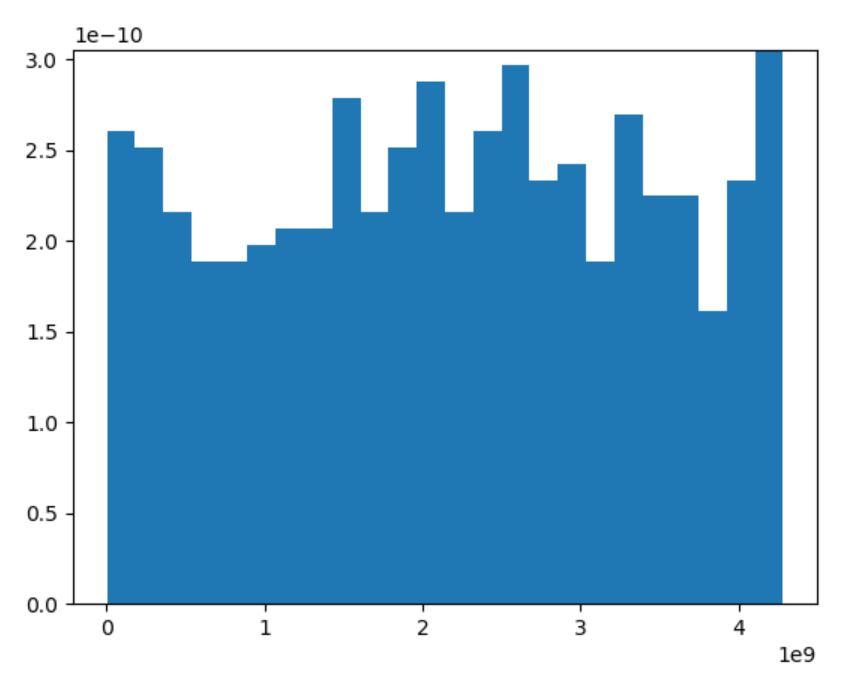# numpy.random.get_state() in Python

• Last Updated : 26 Nov, 2021

With the help of numpy.random.get_state() method, we can get the internal state of a generator and return the tuple by using this method.

Syntax : numpy.random.get_state()

Attention geek! Strengthen your foundations with the Python Programming Foundation Course and learn the basics.

To begin with, your interview preparations Enhance your Data Structures concepts with the Python DS Course. And to begin with your Machine Learning Journey, join the Machine Learning - Basic Level Course

Return : Return the tuple having {tuple(str, ndarray of 624 units, int, int, float), dict}

Example #1 :

In this example we can see that by using numpy.random.get_state() method, we are able to get the tuple having internal state of a generator and return the tuple by using this method.

## Python3

 `# import numpy and get_state``import` `numpy as np` `# Using get_state() method``gfg ``=` `np.random.get_state()[``0``]` `print``(gfg)`

Output :

MT19937

Example #2 :

## Python3

 `# import numpy and get_state``import` `numpy as np``import` `matplotlib.pyplot as plt` `# Using get_state() method``gfg ``=` `np.random.get_state()[``1``]` `count, bins, ignored ``=` `plt.hist(gfg, ``24``, density ``=` `True``)``plt.show()`

Output :My Personal Notes arrow_drop_up